MORE IN Mechanics of Materials
VTU Mechanical Engineering (Semester 3)
Mechanics of Materials
June 2012
Total marks: --
Total time: --
INSTRUCTIONS
(1) Assume appropriate data and state your reasons
(2) Marks are given to the right of every question
(3) Draw neat diagrams wherever necessary

1 (a) Define:
poission's ration;
Modulus of rigidity
Bulk modulus
factor of safety
4 M
1 (b) show that the extension produced due to self weight of a bar of uniform cross section fixed at one end and suspected vertically is equal to half the extension produced by a load equal to self weight applied at the free end.
8 M
1 (c) A member ABCD is subjected to point loads p1,p2,p3 and p4as shown in fig.Q1?.calculate the force p2 necessary for equlibrium if p1=45k,p3=450k and p4=130kN.Determine the total elogation of the member assuming the modulus of elasticity to be 2.1 ×105N/mm2.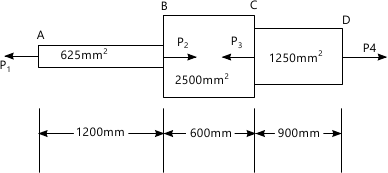8 M

2 (a) Define volumetric strain .A bar of uniform reactangular section of area A is subjected to an axial load P.show that the strain is give by _{\varepsilon v}=\dfrac{P}{AE}\left [ 1-\dfrac{2}{m} \right ],where E is the Young modulus and \frac{1}{m} is the poisson's ratio
4 M
2 (b) The modulus of rigidity of a material is 0.8× ;105N/mm2 when a 6mm ×6mm rod of this material was subjected to an axial pull of 3600 N,it was found the the lateral dimension of the rod chnaged to 5.99991mm.find the poission's ratio and the modulus of elasticity
6 M
2 (c) A horizontal of rigid bar AB weighing 200kN is hung by three vertical rods ,each of 1m lenghth and 500mm2mm2 in cross section as shown in figure fig .Q.2 ?.the central rod of steel and the outer rods are copper .if the tempreture rise is 40°C.estimate the loads cariied by each rod and by flow much the load will descend.
Take:
Es=200 GN/m2
Ec=100 GN/m2
Ls=1.2 ×10-5/°C
Lc=1.8×10-5/°C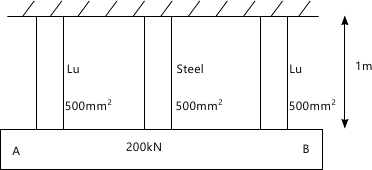10 M

3 (a) A rectangular bar is subjected to two direct stress ?x and ?y in two mutually perpendicular direction .prove that the normal stress(?n) and shear stress(??)on an oblique palne which is inclined at an angle ? with the axis of minor stress are given by:_{\sigma n}= \dfrac{_{\sigma x+\sigma y}}{2}+\dfrac{_{\sigma x-\sigma y}}{2}cos2\Theta and \tau =\dfrac{_{\sigma x-\sigma y}}{2}sin2\Theta
8 M
3 (b) A point in strained material is subjected to stress shown in Fig.Q.3(b).Using Mohr;s circle ,determine the normal and tangential stresses across the obloqiue plane .check the answer analytically.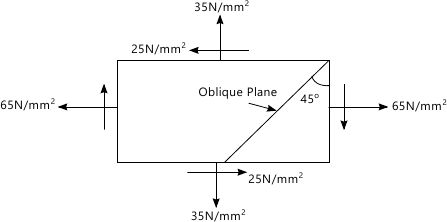12 M

4 (a) A cantillve of uniform section carries a point load at the free end .Find the strain enegy stored by the cantilever and hence calculate the deflaction at the free end.
6 M
4 (b) Calculate the
change in diameter
change in lenghth and
change in volume of a thin cylindical shell 1000mm thick and 5m long when subjected to an internal pressure of 3 N/mm2.take the value of E=2×105 mm2 and 1/m=0.3
6 M
4 (c) A pressure vesste with outer and inner diameter of 400 mm and 320 mm respectively is subjected to an external pressure of 8MPa .determine the circumfrereal stress induced at inner and outer surfaces .prove that the longitudianal strain is constant through our the cylinder
8 M

5 (a) Define
shear force,br>Bending moment
point of cartraflexure
3 M
5 (b) Draw shear force and bending moment diagrams for a simply supported beam sbjected to couple at midspan ,as shown in fig.Q.5(b).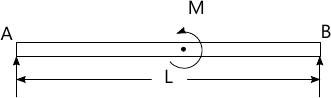5 M
5 (c) A cantilever beam is loaded as shown in fig.Q.5(c) .draw the shear and bending moment diagrams ,for the beam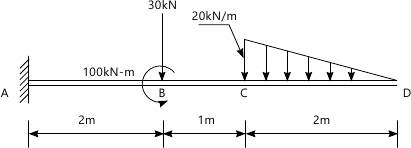12 M

6 (a) A simply supported beam of span 5m has a cross section 150mm×250mm.if the permisable stress is 10 N/mm2,find
Maximum intensity of uniformly distributed load can carry
The maximum concentrated load P applied at 2m from one end can carry.
10 M
6 (b) Prove that the maximum shear stress in circular section of a beam is \dfrac{4}{3} times the average shear stress
10 M

7 (a) Find the expression for the slope and deflation of a cantilefever of length L.Carrying uniformaly distributed load over the whole length
8 M
7 (b) A horizontal girder of steel having uniform section is 14m long is simply supported at its end .It carries concentrated loads of 120kN and 80kN at two points 3m and 4.5m from the two ends respectively .'I' for the section of the gider is 16 ×108 mm4 and E8=210 kN/mm2.Calculate the defection of the girder at ponits under the two loads .also find the maximum deflection
12 M

8 (a) Derive the torison with usual notation .State the assumption made in the derivation
10 M
8 (b) Derive an expression for Eular's buckling load for a long column having one end fixed and other end hinged .State the assumption made in the derivation
10 M

More question papers from Mechanics of Materials